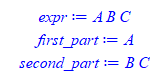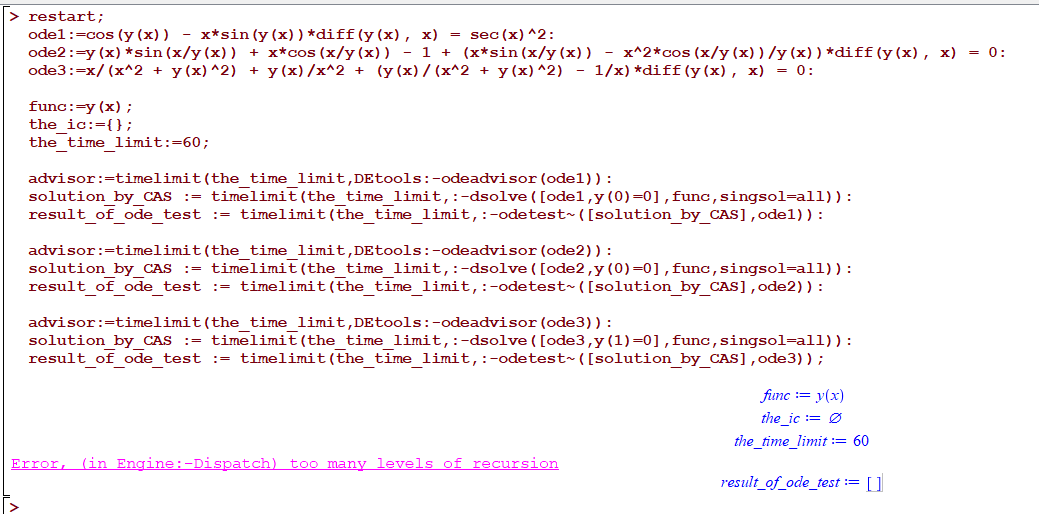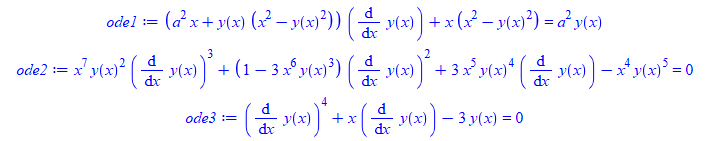## How can I find the solution of an ODE with giving ...

Hi,

I am trying to find q(t) from this

ode1:=-diff(q(t), t) - 1.851851852*10^(-7)*q(t)*(2.042885233*10^10 - 3.517396152*10^18*(0.00001474262739*cos((1000*sqrt(1122)*t)/33) + 0.00001474262739*sin((1000*sqrt(1122)*t)/33))^2) + 9.259259260*10^(-7);

with initial condition

ics := q(0) = 2.45*10^(-12)

using

dsolve({ode1,ics})

but I cannot get the full solution. Is there any way I could find q(t)?

Thanks,

Baharm31

This is the source:

ode1.mw

## Why is Maple 2020.1 so slow?...

Whay the command:  123! + 1 takes significantly more time than 123! only??? Moreover time(123!+1) and time(123!) are very similar and fast.

I am using Worksheet GUI.

## how to access local object variables from a proc()...

I do not understand what I am doing wrong. I have an Object, with private variable call hint. This private variable can be seen only by other proc's inside the object as expected.

Now inside one of those other proc's, I nested a small local proc inside it.

What I want is to access the object private variable from inside this small local proc nested inside.

But not able to. I tried different combinations and nothing is working.  Here is one of many attempts.

```restart;
module my_class()
option object;
local hint::string:="X"; #private variable to the object

export foo::static:=proc(o::my_class) #can be seen from outside
local boo; #local proc inside proc foo

boo:=proc(o::my_class) #local proc
print("inside boo()");
print(o:-hint);
end proc;

print("in foo(), calling local proc boo()");
print("hint is ",o:-hint); #works OK
o:-foo:-boo(o); #tried also o:-boo(o) and boo(o)
end proc;
end module;
```

called it as

```o:=Object(my_class);
o:-foo(o)
```

Error, (in my_class:-foo) module `my_class` does not export `boo`

Also tried different combinations but so far nothing worked. For example, I tried making the nested proc static, but that did not help:

```restart;
module my_class()
option object;
local hint::string:="X"; #private variable to the object

export foo::static:=proc(o::my_class) #can be seen from outside
local boo; #local proc inside proc foo

boo::static:=proc(o::my_class) #local proc, static
print("inside boo()");
print(o:-hint);
end proc;

print("in foo(), calling local proc boo()");
print("hint is ",o:-hint); #works OK
boo(o);
end proc;
end module;

o:=Object(my_class);
o:-foo(o)
```

Error, (in boo) module `my_class` does not export `hint`

But this error do not show up, when I made boo local to the object itself (vs. local to the proc). As follows, where the nested boo() proc is moved one level higher.

```restart;
module my_class()
option object;
local hint::string:="X"; #private variable to the object

export foo::static:=proc(o::my_class) #can be seen from outside
print("in foo(), calling local proc boo()");
print("hint is ",o:-hint); #works OK
o:-boo(o);
end proc;

local boo:=proc(o::my_class) #local proc to the object
print("inside boo()");
print(o:-hint);
end proc;
end module;
```

now

```o:=Object(my_class);
o:-foo(o)
```

works. No problem and no error.

How does one call a local proc to an object? Similar to how one does it in normal non-object proc's?

Are local proc's inside object proc's allowed? Or for an Object, only one level of nesting is allowed?

Maple 2020.1

## easy way to break expression of type `*` to two pa...

In Maple, an expression of type `*` can have many operands. I need to break it to product of two operands only.

For example given, expr:=A*B*C, I want first_part to be A and second_part to B*C

The way I do it now is clumsy.

I first check if expression is of type `*`, then use first_part :=op(1,expr) to find the first term, then divide the expression by that first term to find the second part. second_part:=expr/first_part

second_part:=op(2..nops(expr)) does not exaclty works, since it gives me B,C instead of B*C.

What would be a good way to do this? Notice that expr:=-A is actually a `*`, where first_part:=-1 and second_part:=A. Which is OK.

```restart;
expr         := A*B*C;
first_part   := op(1,expr);
second_part  := expr/first_part;
```## orthogonal affinity to oblique line and k ratio...

How to draw a circle transfomed by orthogonal affinity to oblique line and k ration ? Thank you.

## Dot product of a free vector in cartesian and root...

When I create a rooted vector

```v1:=RootedVector(root=[1,Pi/4,0],[0,0,1],spherical[r,theta,phi])
```

and a free vector

`v2:=Vector([0,2,1],cartesian[x,y,z])`

and dot product them

`v1.v2`

it gives me 2.

But if I change v1 to

`v1:=RootedVector(root=[1,Pi/6,0],[0,0,1],spherical[r,theta,phi])`

the product of v1.v2 gives me an error

"..cannot combine two rooted vectors with different points of origin"

Can anyone explain me the problem?

Here is what I understand:

In spherical coordinates the unit vector e_r, e_th, e_ph are functions of theta and phi. So when I call root=[1,Pi/4,0] that means r=1, theta=Pi/4 and phi=0. This uniquely defines the unit vectors in spherical coordinates and r places the vector away in the e_r direction. The [0,0,1] then attaches a vector to the point r=1, theta=Pi/4 and phi=0  with only 1 unit along the e_ph direction.

What I don't understand is the concept of origin with the free vector and the rooted vector. How changing the theta from Pi/4 to Pi/6 makes the origin "different"?

Edit: This is part of the VectorCalculus pacakge.

## solve numerical the ODEs...

Hi:

How can I solve this system with numerical method:

eq1 := diff(f_n(zeta), zeta\$2)-h_n(zeta)*(diff(f_n(zeta), zeta))-f_n(zeta)^2+g_n(zeta)^2 = 0;
eq2 := diff(g_n(zeta), zeta\$2)-h_n(zeta)*(diff(g_n(zeta), zeta))-2*f_n(zeta)*g_n(zeta) = 0;
eq3 := 2*f_n(zeta)+diff(h_n(zeta), zeta) = 0;
ics := f_n(0) = 0, f_n(10) = 0, g_n(0) = 1, g_n(10) = 0, h_n(0) = 0;
tnx...

## How to avoid memory exhaustion when using Grid?...

Hello

Maple is dangerously close to using all available memory on my Linux machine by doing a calculation using  Grid:-Seq (Map, ..).   Is there a way to control the amount of memory used? Is numcpus a good option?   Or tasksize?

I expect that Maple will use a large amount of memory since the calculations are performed over a rather large list of sets.

Many thanks.

Ed

[Moderator's note: The original version of this Question mentioned using both Grid and Threads. The following 5 Replys will not make sense without knowing that Threads was mentioned.

## how do I plot multiple graph in a single plot?...

Hello

I am having equation y(x) in 5 variable c1, c2, A, R and x.

I am not able to plot graph in x and y(x) for A=1, c1 = 2.3, c2 = 2.4 and R=0,2 5,9.

Range of x: -1..1

Caption :graph of y(x) at different value of R.

Legend: R=0, R=1, R=2, R=3.

my equation is## Spline Differentiation...

I have the numeric solution of differential equation and interpolate the data points with a spline of third degree. Is there a way of differentiating the spline as a whole? Differentiating every single polynom seems awkward.

## Simulate murmuration...

Help me please.  How can i simulate murmuration in maple.

## random error Error, (in Engine:-Dispatch) too many...

it took a while to track this. When I run these 3 ode's in this sequence, Maple gives

Error, (in Engine:-Dispatch) too many levels of recursion

and it has to be this sequence.  I also found I might have to run the code 2 or 3 times to get this error if it does not show first time.

Will show a movie below also.

Here is the code

```restart;
ode1:=cos(y(x)) - x*sin(y(x))*diff(y(x), x) = sec(x)^2:
ode2:=y(x)*sin(x/y(x)) + x*cos(x/y(x)) - 1 + (x*sin(x/y(x)) - x^2*cos(x/y(x))/y(x))*diff(y(x), x) = 0:
ode3:=x/(x^2 + y(x)^2) + y(x)/x^2 + (y(x)/(x^2 + y(x)^2) - 1/x)*diff(y(x), x) = 0:

func:=y(x);
the_ic:={};
the_time_limit:=60;

solution_by_CAS := timelimit(the_time_limit,:-dsolve([ode1,y(0)=0],func,singsol=all)):
result_of_ode_test := timelimit(the_time_limit,:-odetest~([solution_by_CAS],ode1)):

solution_by_CAS := timelimit(the_time_limit,:-dsolve([ode2,y(0)=0],func,singsol=all)):
result_of_ode_test := timelimit(the_time_limit,:-odetest~([solution_by_CAS],ode2)):

solution_by_CAS := timelimit(the_time_limit,:-dsolve([ode3,y(1)=0],func,singsol=all)):
result_of_ode_test := timelimit(the_time_limit,:-odetest~([solution_by_CAS],ode3));
```And here is a movie (this time it happend on second run) sometimes it happens on first run. so it is random.Maple 2020.1

Why does it happen and is there a work around?

## solve the ode system...

Hi:

How can I solve the below ODE system in Maple?

sys_ode := diff(F0(zeta), zeta, zeta)-b^2*F0(zeta)+G0(zeta)^2 = 0, diff(G0(zeta), zeta, zeta)-b^2*G0(zeta) = 0, 2*F0(zeta)+diff(H0(zeta), zeta) = 0:
ics := F0(0) = 0, G0(0) = 1, H0(0) = 0, F0(infinity) = 0, G0(infinity) = 0:

Note: b is a constant.

tnx...

## few errors using dsolve and and odetest...

Noticed few errors generated on some ode's. I do not know if these are expected or known, I thought may be someone might want to check them.

```restart;
ode1:=(a^2*x+y(x)*(x^2-y(x)^2))*diff(y(x),x)+x*(x^2-y(x)^2) = a^2*y(x);
ode2:=x^7*y(x)^2*diff(y(x),x)^3+(1-3*x^6*y(x)^3)*diff(y(x),x)^2+3*x^5*y(x)^4*diff(y(x),x)-x^4*y(x)^5 = 0;
ode3:=diff(y(x),x)^4+x*diff(y(x),x)-3*y(x) = 0;
````sol:=timelimit(60,:-dsolve(ode1,y(x))):`

Error, (in dsolve) numeric exception: division by zero

```sol:=timelimit(60,:-dsolve(ode2,y(x))):
timelimit(60,:-odetest([sol],ode2))
```

Error, (in IntegrationTools:-Indefinite:-AlgebraicFunction) argument is not an algebraic

```sol:=timelimit(60,:-dsolve(ode3,y(x))):
timelimit(60,:-odetest(sol,ode3));
timelimit(60,:-odetest(sol,ode3))
```

Error, (in odetest) received not an ODE w.r.t x(_T)
Error, (in odetest) received not an ODE w.r.t y(_T)

This last one is only due to how the solution is given. So I do not think it is a real problem.

Maple 2020.1, Physics 708

windows 10

Maple Worksheet - Error
Failed to load the worksheet /maplenet/convert/check.mw .